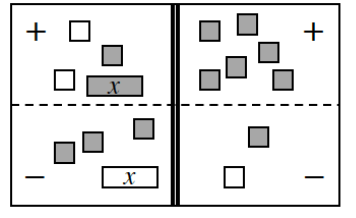### Home > INT1 > Chapter A > Lesson A.1.6 > ProblemA-61

A-61.

For the following equations, solve for x. Record your work by showing each step, and also record the simplified expression that remains after each move. Be sure to check your answer, if possible.

1.Move all tiles in the subtraction region to the addition region by flipping the tiles from positive to negative, and remove any zero pairs. Write the equation represented.

$2x − 4 = 6$
Solve for $x$.

$2x − 4 = 6 \\\quad+4 \:\: +4$

$x = 5$

1. $3x+7=−x−1$

Add $x$ to both sides and subtract $7$ from both sides.

1. Use a diagram of an Equation Map or some other method to explain why $−\left(x − 3\right) = −x + 3$.

Refer to part (a).